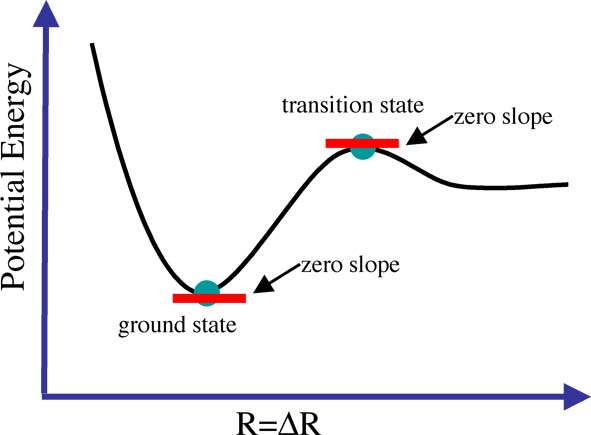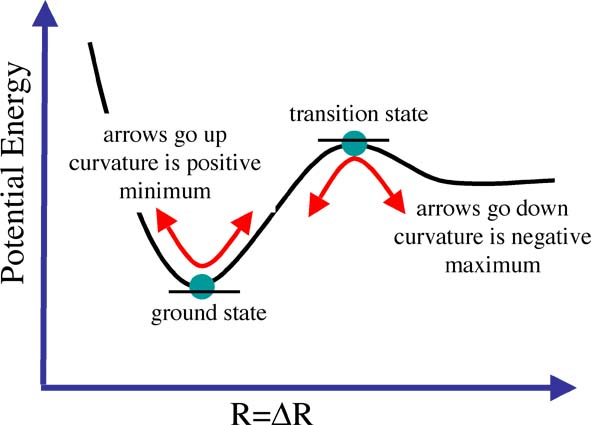## Vibrational Analysis and Confirming Minima

I hope you remember from your pre-university days that while the first derivative of a function tells us the slope, it doesn't tell us if we are at a maximum or a minimum point! For example consider the case where there is a barrier to dissociation in our one dimensional example, as shown below, both the maximum of the barrier (the transition state) and the minimum (the ground state) have a slope of zero.We will have to take the second derivative, if the second derivative is positive we have a minimum and if the second derivative is negative we have a maximum. The second derivative gives the curvature of the function, how this works is shown in the figure below.
When we carry out a frequency or vibrational analysis we are doing two things at once. The frequency analysis is essentially the second derivative of the potential energy surface, if the frequencies are all positive then we have a minimum, if one of them is negative we have a transition state, and if any more are negative then we have failed to find a critical point and the optimisation has not completed or has failed.

The frequency analysis has another important role to play, which you can guess from the name "frequency analysis", this calculation also provides the IR and Raman modes (or frequencies) to compare with experiment.

This means that the curvature, or second derivative of the potential energy surface is closely related to the vibrations a molecule undertakes.

### Now we are ready to look at the vibrations of the NH3 molecule and to confirm we have minium and not a transition state structure.

When you are ready close the molecule window and move onto the next step